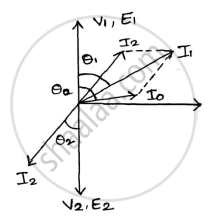# A Single Phase Transformer Has 1000 Turns on the Primary and 200 Turns on the Secondary. the No Load Current is 3a - Basic Electrical and Electronics Engineering

Sum

A single phase transformer has 1000 turns on the primary and 200 turns on the secondary. The no load current is 3A at a power factor of 0.2 lag and the secondary current is 280A at a power factor of 0.8 lag. Neglect Rand X2 calculate (1) Magnetizing component and loss component of no load current. (2) primary current (3) input power factor .Draw phasor diagram showing all the currents.

#### Solution

I_P/I_S=N_S/N_P

Therefore, I_P=N_S/N_PxxI_S=200/1000xx280=56A

cos𝜑2=0.8 cos𝜑0=0.2 sin𝜑2=0.6 sin𝜑0=0.98
solve for horizontal and vertical components
𝐼1𝑐𝑜𝑠𝜑1=𝐼2𝑐𝑜𝑠𝜑2+𝐼0𝑐𝑜𝑠𝜑0 =(56×0.8)+(3×0.2)=45.4𝐴
𝐼1𝑠𝑖𝑛𝜑1=𝐼2𝑠𝑖𝑛𝜑2+𝐼0𝑠𝑖𝑛𝜑0 =(56×0.6)+(3×0.98) = 36.54A
𝐼1=sqrt(45.4^2+36.54^2)=58.3𝐴
𝑰𝝁=𝑰𝟎𝒔𝒊𝒏𝝋𝟎=(𝟑×𝟎.𝟔)=𝟏.𝟖Atan𝜑1 (36.54)/(45.4)= 0.805

𝛗𝟏=𝟑𝟖°
Power factor cos𝝋𝟏=𝒄𝒐𝒔𝟑𝟖°=𝟎.𝟕𝟖 lagging.

Concept: Construction of Single Phase Transformer
Is there an error in this question or solution?
2018-2019 (December) CBCGS

Share